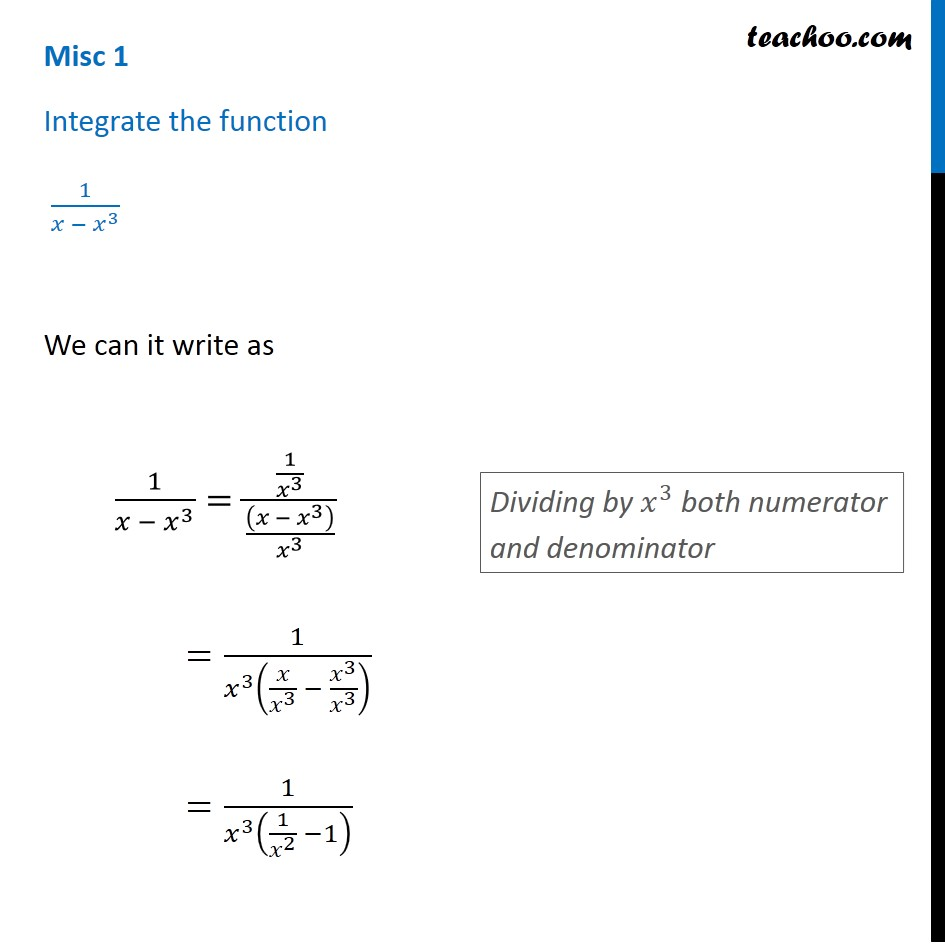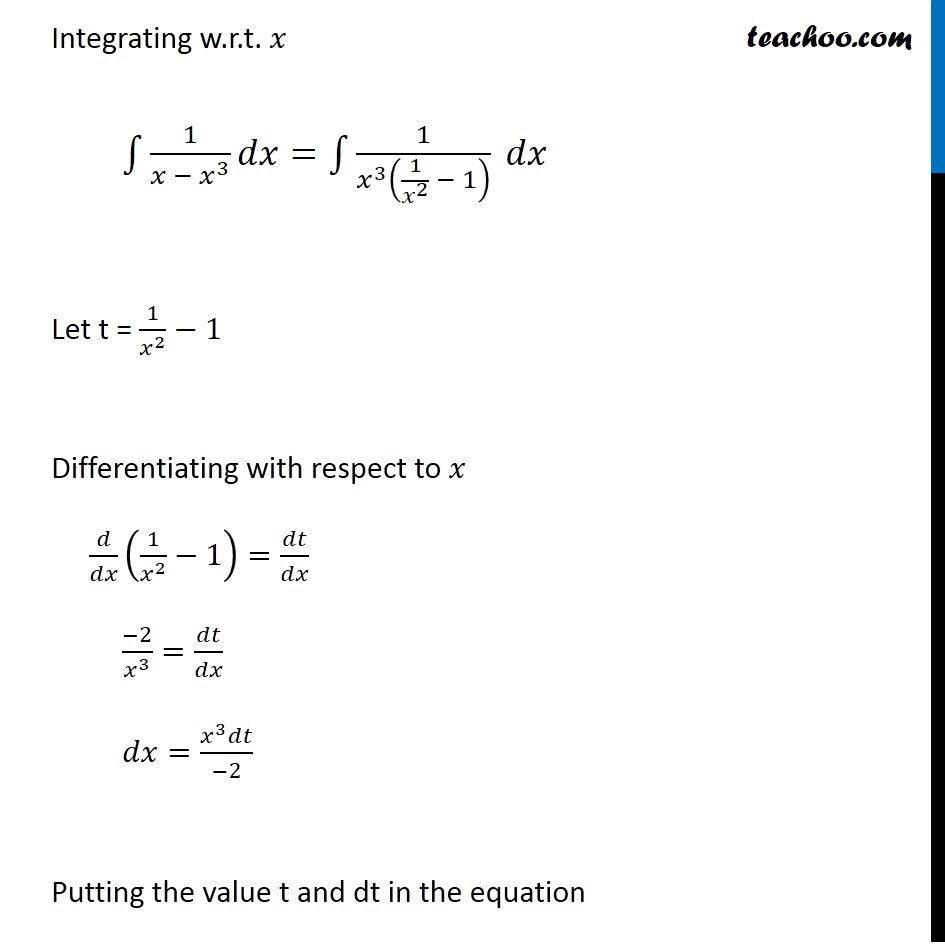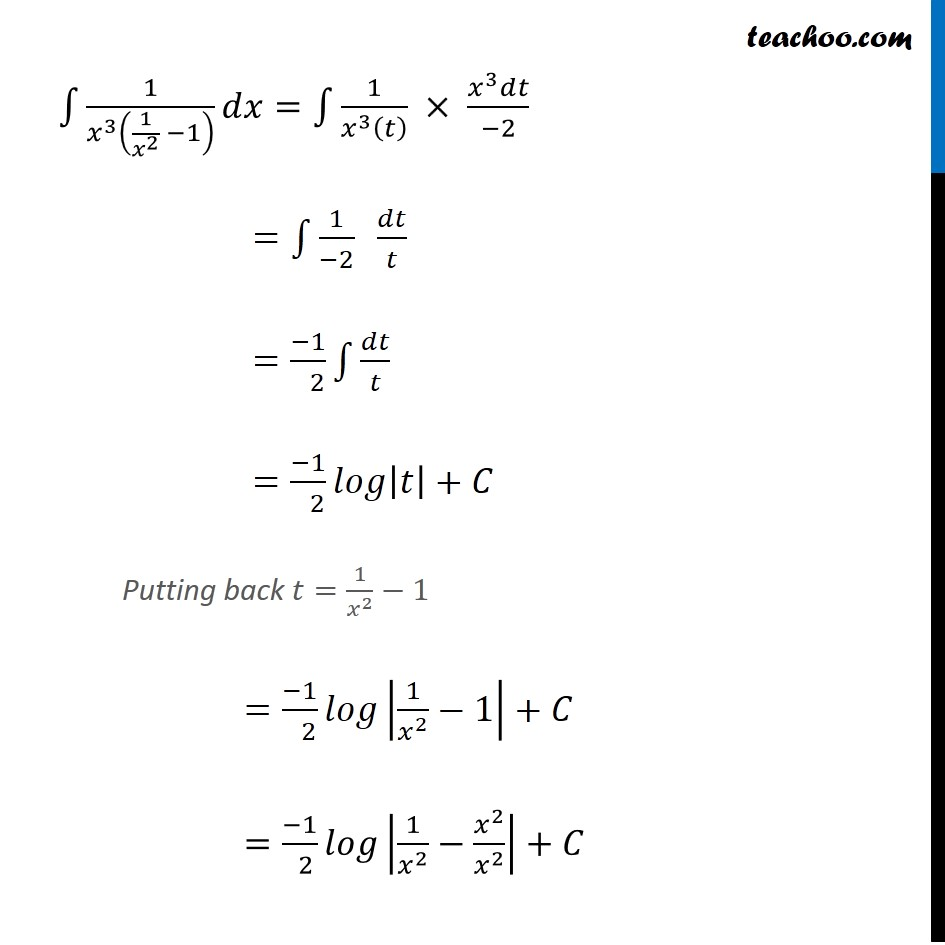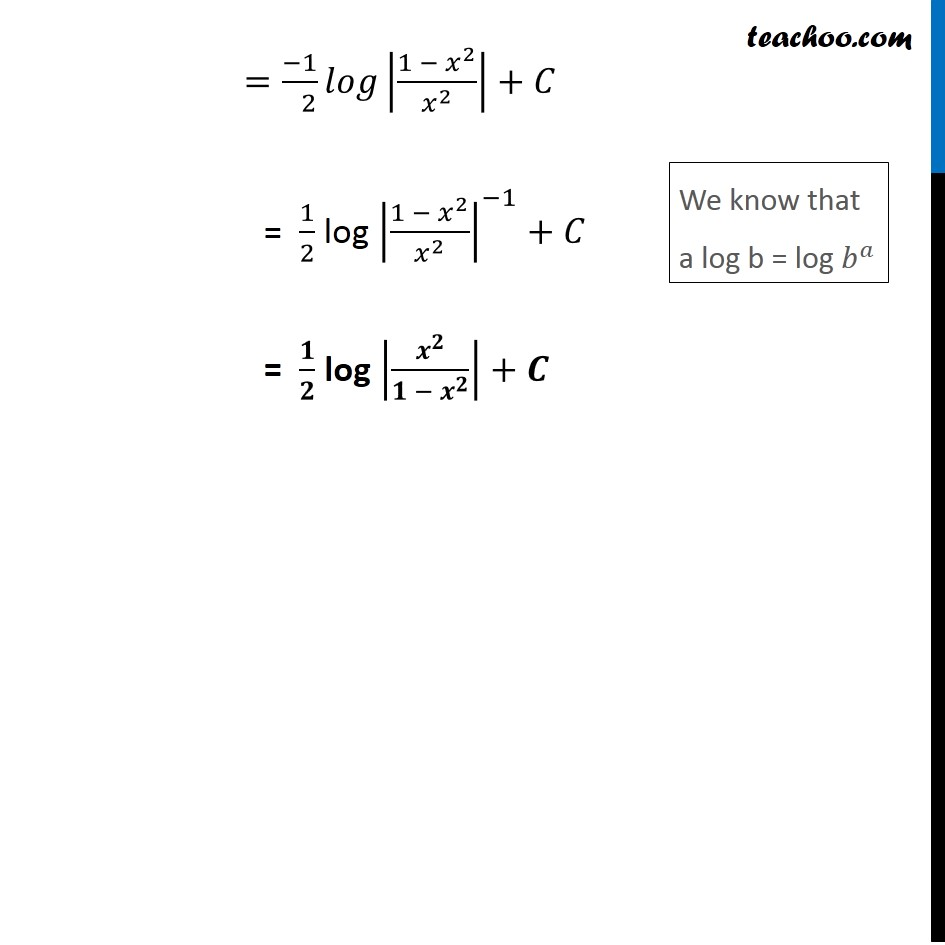1. Chapter 7 Class 12 Integrals (Term 2)
2. Serial order wise
3. Miscellaneous

Transcript

Misc 1 Integrate the function 1/(𝑥 − 𝑥^3 ) We can it write as 1/(𝑥 − 𝑥^3 )=(1/𝑥^3 )/(((𝑥 − 𝑥^3 ))/𝑥^3 ) =1/(𝑥^3 (𝑥/𝑥^3 − 𝑥^3/𝑥^3 ) ) =1/(𝑥^3 (1/𝑥^2 −1) ) Dividing by 𝑥^3 both numerator and denominator Integrating w.r.t. 𝑥 ∫1▒〖1/(𝑥 − 𝑥^3 ) 𝑑𝑥〗=∫1▒1/(𝑥^3 (1/𝑥^2 − 1) ) 𝑑𝑥 Let t = 1/𝑥^2 −1 Differentiating with respect to 𝑥 𝑑/𝑑𝑥 (1/𝑥^2 −1)=𝑑𝑡/𝑑𝑥 (−2)/𝑥^3 =𝑑𝑡/𝑑𝑥 𝑑𝑥=(𝑥^3 𝑑𝑡)/(−2) Putting the value t and dt in the equation ∫1▒〖1/(𝑥^3 (1/𝑥^2 −1) ) 𝑑𝑥〗=∫1▒〖1/(𝑥^3 (𝑡) ) × (𝑥^3 𝑑𝑡)/(−2)〗 =∫1▒〖1/(−2) 𝑑𝑡/𝑡〗 =(−1)/( 2) ∫1▒𝑑𝑡/𝑡 =(−1)/( 2) 𝑙𝑜𝑔|𝑡|+𝐶 Putting back 𝑡=1/𝑥^2 −1 =(−1)/( 2) 𝑙𝑜𝑔|1/𝑥^2 −1|+𝐶 =(−1)/( 2) 𝑙𝑜𝑔|1/𝑥^2 −𝑥^2/𝑥^2 |+𝐶 =(−1)/( 2) 𝑙𝑜𝑔|(1 − 𝑥^2)/𝑥^2 |+𝐶 = 1/2 log |(1 − 𝑥^2)/𝑥^2 |^(−1)+𝐶 = 𝟏/𝟐 log |𝒙^𝟐/(𝟏 − 𝒙^𝟐 )|+𝑪 We know that a log b = log 𝑏^𝑎

Miscellaneous